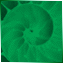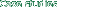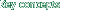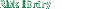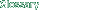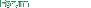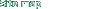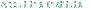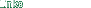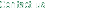Risk Library
 Documents by Author
 Group of Thirty (G30)
 Appendix I: Working Papers Global Deriva...
 I. Credit Risk Measurement
 Credit Exposure on a Derivatives
 Credit Exposure on a Portfolio of Deriva...
 The Probability of Default and Expected ...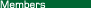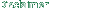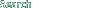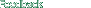### Credit Exposure on a Derivatives

 Recommendation 10: Measuring Credit Exposure Dealers and end-users should measure credit exposure on derivatives in two ways: Current exposure, which is the replacement cost of derivatives transactions, that is, their market value. Potential exposure, which is an estimate of the future replacement cost of derivatives transactions. It should be calculated using probability analysis based upon broad confidence intervals (e.g., two standard deviations) over the remaining terms of the transactions. Credit risk fluctuates over time with the variables that determine the value of the underlying contract. In assessing credit risk, one needs to ask two questions: If a counterparty was to default today, what would it cost to replace the derivatives transaction (i.e., what is the current exposure)? If a counterparty defaults at some point in the future, what is a reasonable estimate of the potential replacement cost (i.e., what is the potential exposure)? The first question is fairly straightforward, as it simply asks for the current mark-to-market price of the underlying contract. This price can be positive or negative. As an example, consider a \$100-million, 5-year swap executed at the prevailing market condition in which one party pays a fixed rate of 6.00% and the other party pays a floating rate of LIBOR. The mark-to-market value of such a swap (i.e., its current replacement cost) is zero at the time the swap is executed; however, as time passes and interest rates move, the mark-to-market value of the swap will also move. Suppose that in six months, the prevailing swap rate for a 4.5-year swap is 5.50%. If the counterparty paying the fixed rate of 6.00% defaults, the nondefaulting counterparty receiving the fixed rate (and paying the floating rate) will be forced to replace it with a 5.50% swap and will thereby suffer a replacement cost equal to 0.50% per annum for the remaining 4.5 years plus whatever net unpaid swap payment has accrued. The second question is more difficult to answer in that it asks for an assessment of what the replacement cost could be in the future if the variables that determine the value of the underlying contract were to move adversely. Returning to the swap example, the value for the \$100-million, 5-year swap could attain a significant positive or negative value over the life of the swap. The extent to which the value of the swap could become positive is the potential exposure. Dealers use Monte Carlo or historical simulation studies, option valuation models, and other statistical techniques to assess potential exposure. The analysis generally involves modeling the volatility of the underlying variables (such as interest rates, exchange rates, equity prices, or commodity prices) and the effect of movements of these variables on the value of the derivatives contract. These techniques are often used to generate two measures of potential exposure: expected exposure; and maximum or "worst case" exposure. Expected exposure at any point during the life of the swap is the mean of all possible probability-weighted replacement costs, where the replacement cost in any outcome is equal to the mark-to-market present value if positive and zero if negative. Loosely speaking, expected exposure is the best estimate of the present value of the positive exposure that is likely to materialize. As such, expected exposure is an important measure in derivatives dealers´ capital-allocation and pricing decisions. The maximum potential exposure is calculated as an estimate of "worst case" exposure at any point in time. These calculations are based on adverse movements in the underlying variables that are extreme enough that they are unlikely to be exceeded. For example, if two standard deviations in a one-tail test are used to calculate maximum potential exposure, there is statistically only a 2.5% chance the actual exposure will be greater than the calculated maximum exposure. This "worst case" exposure is important in assessing the maximum amount that could possibly be at risk to a given counterparty. As such, it is important in the dealer´s credit-allocation decisions. The expected and maximum potential exposure profiles for an interest rate swap executed at current market levels is shown in Figure 1. The "hump-back" profile is due to the offsetting effects that the passage of time has on the magnitude of the potential movement in the underlying variables, and the number of cash flows that need to be replaced if a default should occur. The first effect of the passage of time on potential exposure is that it increases the probability that the underlying variable will drift substantially away from its initial value. This "diffusion effect" is determined by the volatility of the underlying variable and its other stochastic properties. The second effect of the passage of time, called the "amortization effect," is the reduction in the number of years of cash flows that need to be replaced. The offsetting influences of the diffusion effect and the amortization effect create the concave shape in Figure 1 as the passage of time increases the potential for large per annum replacement costs, but reduces the number of years of cash flows that need to be replaced.Figure 1 can be deduced by looking at the present value of the replacement cost if a default occurs immediately after the swap is executed and immediately prior to its maturity. If a default occurs immediately after the swap is executed, five years of cash flows will need to be replaced but it is unlikely that the swap rate will have moved very far from its initial level in such a brief period. Consequently, the expected and maximum potential exposures are low because the diffusion effect is low. At the other extreme, if a default occurs just prior to the swap´s last payment date, the market swap rate could be substantially different from its initial level, but because only one semiannual cash flow will need to be replaced, the expected and maximum potential exposures are low. The peak exposure (top of the "hump") occurs at an intermediate point during the swap´s life when sufficient time has passed for the per-annum replacement cost to be high, and sufficient time still remains for the impact of a high per annum replacement cost to be meaningful. The potential exposure profile depends on the cash flow pattern of the underlying asset class and on the type of derivatives transaction. Standard interest rate swaps and other derivatives with periodic payments and no final exchange of principal tend to have the hump-backed shape depicted in Figure 1. If the derivatives transaction calls for a final exchange of principal, as currency swaps do, the potential exposure profile tends to be upward sloping, as in Figure 2. The final exchange of principal increases the importance of the diffusion effect and reduces the amount by which the currency swap amortizes, thereby creating the upward slope of the exposure profile. The exposure profile of purchased options tends to be greater than the credit risk for comparable swaps. Options do not generally have periodic payments but are characterized by an up-front payment of the option premium and a final option payoff payment. Accordingly, the amortization effect is limited to the time decay of the option price and is outweighed by the diffusion effect. That is, the longer the time period, the greater is the scope for movements in the underlying variable, which can generate a large exposure on the option payoff. Moreover, in contrast to swaps, purchased options with the premium paid up front initially create an immediate mark-to-market exposure equal to the option premium. If the option seller defaults immediately, the option buyer must pay another option premium to replace the option even if there has been no movement in the underlying variables.Finally, and again in contrast to swaps, credit risk is always asymmetric for options. The counterparty purchasing the option always has some exposure to the counterparty selling the option, regardless of how far the underlying variables move. The calculation of expected and maximum exposure profiles, such as those in Figures 1 and 2, provides management with an important tool to assess how credit risk can evolve over the life of the transaction. In Figure 1, the maximum exposure profile peaks at about 6.00% of notional amount after two years and declines steadily thereafter. This is known as the "peak maximum exposure." Similarly, the peak expected exposure is the highpoint of the expected exposure profile, or 1.50% of notional amount, and occurs after one year. Another useful summary measurement of the potential exposure profiles is based on the average of the potential exposures over the life of the contract. Returning again to Figure 1, the maximum exposure profile starts at zero and rises rapidly to about 6.00% during the first year and declines steadily to zero over the final four years. The average of these exposures over the life of the contract is 3.25%, known as the "average maximum exposure." The average of the expected exposure profile is calculated in an identical fashion and is 0.90%. As Figure 1 and Figure 2 demonstrate, the maximum ("worst case") exposures on derivatives transactions are typically equal to a relatively small fraction of the notional amount of the contract, expected exposures are much smaller than maximum exposures. This is important to keep in mind when discussing the aggregate notional amount of the derivatives business. Aggregating derivatives transactions by notional amounts indicates the volume and growth of the business, but it provides virtually no information on the underlying credit risk in the system. The analysis of potential exposure profiles enables management to evaluate credit exposures on derivatives transactions on a comparable basis with credit exposures for on-balance-sheet transactions, such as loans. Many institutions use measures such as the peak of the maximum or expected exposure profiles or the average of the maximum exposure profiles for aggregating and comparing credit exposure on derivatives transactions with on-balance-sheet transactions for a given counterparty. The Survey of Industry Practice reveals that approximately two-thirds of dealers use internally developed methods to assess credit risk that are based on maximum exposure calculations using historical volatilities. Of this group, the majority of dealers assign counterparty exposures to individual transactions that are based on tables calculated for a variety of generic transactions (e.g., interest rate swaps, currency swaps, etc.). In the future, most dealers intend to refine this method by calculating the exposure of individual transactions rather than relying on generic tables. Only 22% of dealers surveyed use the Original Exposure Method (OEM), which is a static model of credit risk, and only 12% of dealers surveyed intend to use the OEM in the future. The Survey asked dealers to indicate the initial exposure that would be assigned to a variety of derivatives transactions with varying maturities. The results reveal that most dealers differentiate sharply by the type of transaction and the maturity of the transaction. For instance, the average dealer assigns an initial exposure of about 22% of notional amount for a 3-year currency swap and about 5% of notional amount for a 3-year interest rate swap. This differentiation according to the type of transaction would be expected by the analysis presented above for Figure 1 and Figure 2. The practices of end-users differ from those of dealers. A majority of the end-users indicate that they use some method based on notional amount to measure the exposure of an individual transaction. Twenty-six percent of end-users measure exposure according to the notional amount alone. An additional 39% of end-users measure the exposure as a percent of notional amount, but differentiate by type and maturity of transaction. (This is similar to OEM.) Fourteen percent of end-users measure exposure on a mark-to-market basis.